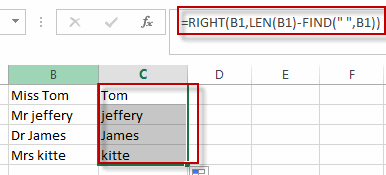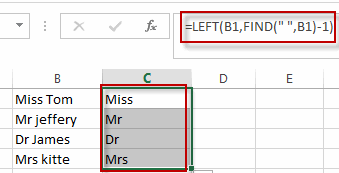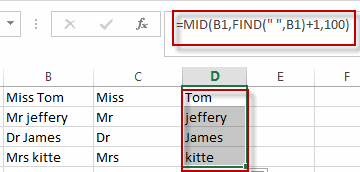# Removing Salutation from Name

This post will guide you how to remove salutations from names in the range of cells in excel. How do I remove the salutation from the names cells with formula in Excel. For example, if you have name salutations and you want to remove those salutations from name strings in excel, how to achieve it. How to split salutation and only names string into separate columns with excel formula.

## Removing Salutation from Name

To remove salutation from names string in excel, you can create a formula based on the RIGHT function, the LEN function and the FIND function.

Assuming that you have a name list in the range of cell B1:B4, and you want to remove all salutations from those cells, you can write down this formula to achieve the result.

`=RIGTH(B1,LEN(B1)-FIND("",B1))`

You just need to type this formula into a blank cell, then press enter key in your keyboard. Then you still need to drag the AutoFill Handler over other cells to apply this formula to remove salutations.

Let’s see the last result:Split salutations and name strings into different columns

If you want to split salutations and name string into different columns, you can extract the salutation string firstly, then extract the rest name string again. You need to create the first formula based on the LEFT function and FIND function to extract salutation string. And you still need to use another formula based on the MID function and the FIND function to get the names strings from those cells. Like these two formulas:

```=LEFT(B1,FIND(" ",B1)-1)

=MID(B1,FIND(" ",B1)+1,100)```

Type the first formula into a blank cell to get the salutation strings, then press enter key and drag the AutoFill Handler over other cells.Type the second formula into a blank cell in a new column to get the name strings, then press enter key and then drag the AutoFill Handler over other cells to apply this formula to extract names.### Related Functions

• Excel LEFT function
The Excel LEFT function returns a substring (a specified number of the characters) from a text string, starting from the leftmost character.The LEFT function is a build-in function in Microsoft Excel and it is categorized as a Text Function.The syntax of the LEFT function is as below:= LEFT(text,[num_chars])…t)…
• Excel FIND function
The Excel FIND function returns the position of the first text string (sub string) within another text string.The syntax of the FIND function is as below:= FIND(find_text, within_text,[start_num])…
• Excel RIGHT function
The Excel RIGHT function returns a substring (a specified number of the characters) from a text string, starting from the rightmost character.The syntax of the RIGHT function is as below:= RIGHT (text,[num_chars])…
• Excel MID function
The Excel MID function returns a substring from a text string at the position that you specify.The syntax of the MID function is as below:= MID (text, start_num, num_chars)…
• Excel LEN function
The Excel LEN function returns the length of a text string (the number of characters in a text string).The syntax of the LEN function is as below:= LEN(text)…
Related Posts

Extract substring In Excel

This post will guide you how to use Excel's MID function is a quick and easy way to extract pieces from your text. Use the Excel formula to extract a substring with MID. Note: If you want to extract just ...

How to Split Cells by the First Space in Texts in Excel

Sometimes we may use space to separate texts to different groups in one cell, if we want to split this cell to multiple columns refer to space, we can implement this via ‘Text to Columns’ feature. But is there any ...

How to Convert Uppercase to Lowercase Except the First Letter in Excel

In excel, words can be entered in uppercase, lowercase or mixed. If we want to convert all uppercase to lowercase (except the first letter in some situations), we can use formula with some letter convert related functions to convert them ...

How to Convert Text to Time in Excel

This post will guide you how to convert time string to time in Excel. Assuming that you have a list of text string in your worksheet, and you wish to convert these time string to standard time format, how to ...

How to Remove Last or Trailing Commas in Excel

This post will guide you how to remove trailing commas from cell in Excel. How do I remove comma character at the end of a cell with a formula in Excel 2013/2016. Remove Trailing Commas if you want to remove ...

How to Convert mmddyy to Date in Excel

This post will guide you how to convert non-standard date formats or text to a standard date in Excel. If you have a date with mmddyy format, and you want to change it as the normal date format as mm/dd/yyyy ...

How to Convert Military Time to Standard Time in Excel

This post will guide you how to convert military time to standard time with a formula in Excel. How do I convert from military time 17:29:30 to regular time 5:29:30PM in Excel.  How do I Convert Standard time to military ...

How to Sort Data by Last Character in Excel

This post will guide you how to sort cells by last character in Excel. How do I sort data by the last character with a formula in Excel. How to sort cells based on the last character with User Defined ...

How to Remove the First/Last Word from Text string in Cell

This post will guide you how to remove the first and the last word from a text string in cells in Excel. How do I use a formula to remove first and last word of a text string in Excel. ...

How to Remove Prefix and Suffix in Given Cells in Excel

This post will guide you how to remove suffix form a range of cells in Excel. How do I remove prefix from text string in cells with a formula in Excel. Remove Prefix of Cells Remove Suffix of Cells Assuming ...

Sidebar